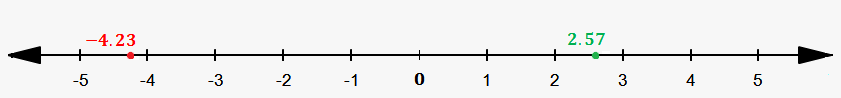# Decimal Number Line

So, where are decimals located on a number line?

For example, where is 2.57 located? We see that this number has integer part 2 and decimal part 0.57.

This means that it is greater than 2 (because there is 2 and something else), but it is less than 2+1=3 because decimal part is always less than 1. Again, we see strong analogy with mixed numbers.

This means that 2.57 lies between 2 and 3.

Let's see how this works with negative numbers.

For example, consider -4.23.

It is less than -4 because there is -4 and something else (which is also negative because minus is in of decimal), but it is greater than -4-1=-5, because, since decimal part is less than 1 then negative decimal part is greater than -1.